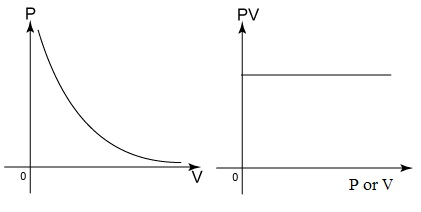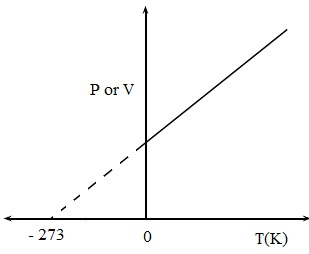#### Gas Law-I, Chemistry tutorial

Introduction:

The kinetic theory was utilized to illustrate the physical nature of the different states of matter and also the changes that take place whenever matter is heated.

We are as familiar that particles in the gas state are in arbitrary motion in all directions and at very high speed with virtually no force of attraction between the particles. The physical behavior of a gas is very much dissimilar from those of the solid and liquid. This physical behavior of gases was investigated via early scientists and that led to the establishment of gas laws named after them. There is a requirement thus to raise the postulates of the kinetic theory to account for the special behavior of gases.

The Kinetic Molecular Theory of Gases:

Two suppositions are made regarding gases in the kinetic theory:

1) There is almost no force of attraction among the gas molecules.

2) Gas molecules move independent of one other.

Such suppositions are only true for the ideal gas. An idea gas is as well frequently termed to as a perfect gas.

Real gases in general don't quite follow the suppositions made for the ideal gas. They comprise what is termed as the kinetic molecular theory. They particularly deal by the gas molecules. These given six statements illustrate the behavior of an ideal gas:

1) A gas comprises of small similar particles termed as molecules moving arbitrarily in all directions colliding with one other and as well by the walls of the containing vessel.

2) There is no force of attraction among the gas molecules.

3) Molecular collisions are perfectly elastic that is, no energy is lost whenever molecules collide with one other or with the container wall.

4) The volume of gas molecules is very small as compared to the container volume.

5) The collisions of gas molecules by the container walls give rise to what is termed as the gas pressure.

6) The temperature of gas is directly proportional to the average kinetic energy of the molecules.

Boyle's Law:

Statement of Boyle's law:

Boyle's law defines that at a constant temperature, the volume of a given mass of gas is inversely proportional to the pressure.

Note: The above is the statement of the law. Boyle's law illustrates the relationship between the pressure and volume of a gas at constant temperature.

Mathematically,

V α 1/P at constant temperature

Or V = Volume and P = Pressure of gas

V = k/p, here k is the constant of proportionality.

PV = k

Boyle's law can as well be stated mathematically as P1V1 = P2V2

P1 is the pressure when gas volume is V1 and P2 is the pressure whenever gas volume is V2.

The above means that the product of the pressure and volume is for all time a constant. What this signifies is that as the gas pressure rises, the volume reduces and vice-versa as long as the temperature is constant.

Boyle's law can as well be represented graphically:Fig: Statement of Boyle's law

Let, consider the equation in the form PV = k

The equation can be represented as P = k/V

The equation above is the mathematical symbolization of the alternative statement of Boyle's law:

The pressure of a particular mass of gas is inversely proportional to the volume given by the temperature is constant.

Illustration:

1) At 25°C and 750mm Hg pressure, the volume of a particular mass of oxygen is 520 cm3. Compute the gas volume if the pressure is increased to 760 mm Hg pressure at constant temperature.

2) A gas occupies a volume of 1.20 dm3 at 25°C and 760 mm Hg pressure. Find out the gas pressure if the gas expands to 2.80 dm3 container at the similar temperature?

1) Assume that P1 = 750 mm Hg and V1 = 520 cm3

P2 = 760 mm Hg and V2 = ?

By employing P1V1 = P2V2

P1V1/P2 = V2

(750 x 520)/760 = V2 = 513 cm3

2) Assume P1 = 760 mm Hg V1 = 1.20 dm3

P2 = ?                  V2 = 2.80 dm3

By employing P1V1 = P2V2

P1V1/V2 = P2

(760 x 1.20)/2.80 = P2 = 326 mm Hg

How kinetic theory explains Boyle's Law:

According to the kinetic molecular theory of gases a raise in pressure of the gas means an increase in the number of molecular collisions per unit area of the containing vessel. We are familiar that for a fixed mass of gas at constant temperature, the number and the average kinetic energy of the molecules remain constant for the number of molecular collisions per unit area of the containing vessel to increase beneath a condition of fixed mass and average kinetic energy of the gas molecules, the area and thus the volume of the containing vessel should reduce. This means that an increase in gas pressure is accompanied via a corresponding decrease in the gas volume. The reverse of the above is right whenever the gas pressure is decreased at constant temperature.

Charles' Law:

Charles' law and the Kelvin temperature scale:

The word temperature is a measure of the degree of coldness or hotness of a body. The Celsius or centigrade (°C) scale is the most utilized temperature scale in scientific work. The volume of matter usually increases with increasing temperature however the increase is most pronounced for gases.

Charles study on gases represent that the volume of a gas increases via 1/273 of its volume at-CPC for each and every °C rise in temperature.

Mathematically stated:

VT = Vo + (T/273) Vo

VT = Volume of the gas at ToC

Vo = Volume of gas at 0oC

According to the equation above, a gas consists of zero volume at - 273°C. This temperature at which the gas volume is theoretically zero (0) is the lowest temperature which can be reached. This is known as the absolute zero temperature. The Kelvin temperature scale symbolized by a capital 'K' has this temperature (-273) as its beginning point and measures temperatures upwards from it.

Remember the relationship between temperature and the average kinetic energy of gas molecules and describe why it is supposed that the average kinetic energy of gas particles at -273°C is zero.

The Celsius and the Kelvin (absolute) scales are associated by the equation.

T(K) = toC + 273

The statement of Charles' law:

Charles' law defines that for a fixed mass of gas, the volume is directly proportional to the absolute temperature given by the pressure remains constant.

V α T(K) or V = KT

V: Volume of the gas

T: Kelvin temperature

Here, k is a constant

V/T = K

Or, V1/T1 = V2/T2, here V1 is the volume at T1 and V2 is the volume at T2

Charles' law can as well be defined in an alternative form if the gas volume is constant and pressure changes with temperature. In this case P α T; P = KT; K = P/T => (P1/T1) = (P2/T2) at constant volume, the pressure of a fixed mass of gas is directly proportional to the Kelvin temperature. Charles' law can as well be represented graphically as illustrated below.Fig: Charles Law

Illustration: At 27°C the volume of mass of helium gas is 1.85 dm3. At what temperature will the volume be 2.25 dm3 whenever pressure is kept constant?

V1 = 1.85 dm3

T1 = 273 + 17 = 300 K

T2 = ?

V2 = 2.25 dm3

By employing the equation: (V1/T1) = (V2/T2)

V1T2 = V2T1;

T2 = V2T1/V1 = (2.25 x 300)/1.85 = 364.9 K

How the Kinetic theory explains Charles' law:

According to the kinetic molecular theory, an increase in the gas temperature leads to increase in the average kinetic energy of the gas molecules. This will yield in more arbitrary motion of the molecules. For a fixed mass of gas, the number of gas molecules is constant and at constant pressure of gas the number of collisions per unit area of the containing vessel is as well constant. The only means for the pressure to remain constant in spite of the increase in the arbitrary motion and more collisions of particles with the wall of the container is for the area and thus the volume of the container to increase. This means that an increase in the temperature of the gas at a constant pressure leads to the corresponding increase in the gas volume.

In the alternative, take the case if the volume of a fixed mass of gas is kept constant and the temperature is raised. At higher temperature, the more arbitrary motion of the gas molecules will lead to more molecular collisions per unit area on the wall of the container and therefore increase in the gas pressure.

The General Gas Equation:

A gas sample is illustrated completely by four quantities: the amount, the temperature, pressure and volume. According to the Boyle's and Charles' laws both pressure and temperature can influence the volume of a gas.

According to the Boyle's law:

V α 1/P and according to Charles law V α T

If both T and P change for a gas sample V α T/P; or V = KT/P

PV = KT; PV/T = K

Thus, PV/T = K (constant)

At times the above is represented as:

P1V1/T1 = P2V2/T2

The above is the general gas equation and is Boyle's and Charles' law combined. For one mole of gas, the constant of proportionality (k) in the general gas equation is the molar gas constant (R) and the general gas equation then reads;

PV = RT. For n moles of gas the equation consists of the form:

PV= nRT.

The value and units of the gas constant R based on the units of P, V and T.

P in atm

V in dm3

T in K

From the general gas equation:

PV = nRT

And R = PV/nT

The value of R is 0.082 atm dm3 K-1 mol-1 when pressure is in atmosphere, volume in dm3 and temperature in Kelvin. However when pressure is in Nm-2, volume in m3 and temperature in Kelvin, the value of R is 8.31 JK-1 mol-1 (that is, Joules per degree Kelvin per mole).

A mass of oxygen gas occupies a volume of 2 x 104 cm3 at a pressure of 1.01 x 105 Nm-2 and temperature of 17°C. Compute the volume if the pressure is raised to 1.35 x 105 Nm-2 and the temperature changed to 43 °C.

P1 = 1.01 x 105 Nm-2     P2 = 1.35 x 105 Nm-2

T1 = 273 + 17 = 300K    T2 = 273 + 43

V1 = 2 x 104 cm3           V2 = ?

By employing the general gas equation in the form

P1V1/T1 = P2V2/T2 and making V2 the subject of the formula

V2 = P1V1T2/T1P2

On replacing the values we get,

V2 = (1.01 x 105 x 2 x 104 x 316)/(300 x 1.35 x 105) = 1.58 x 104 cm3

Tutorsglobe: A way to secure high grade in your curriculum (Online Tutoring)